Anzeige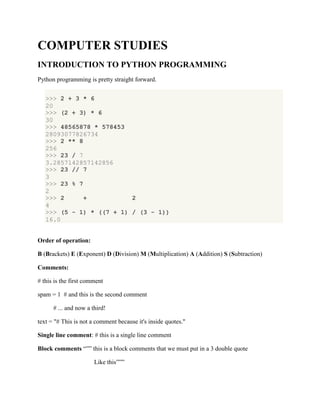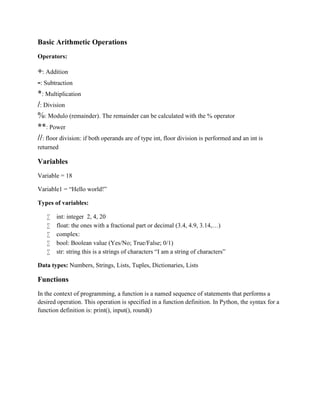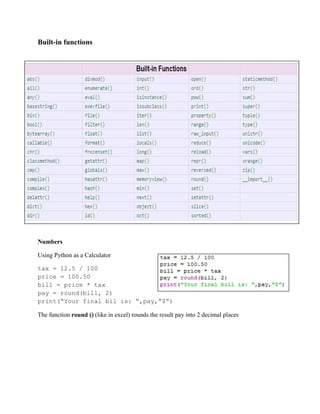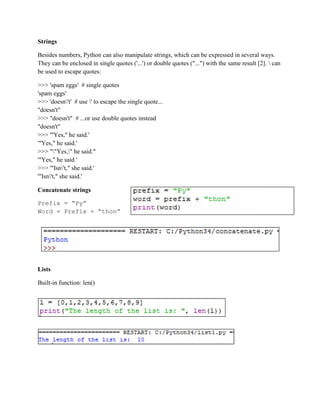Anzeige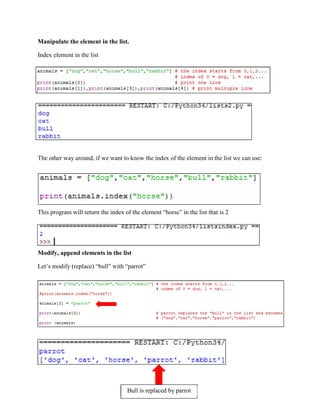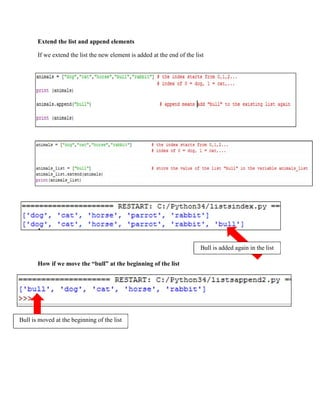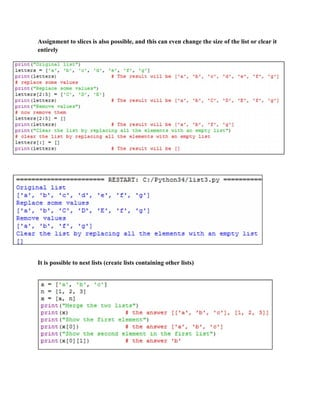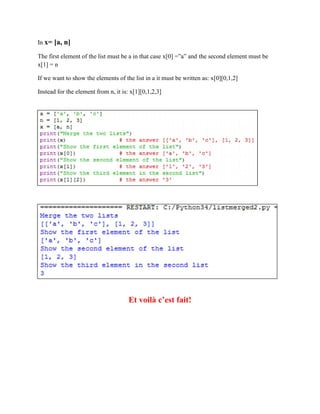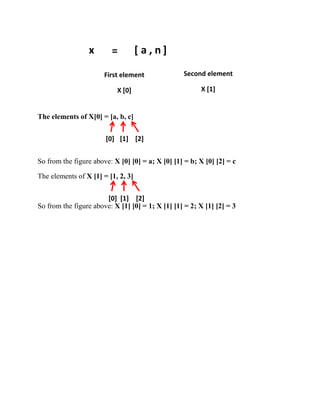Anzeige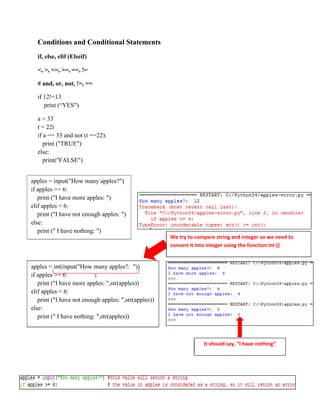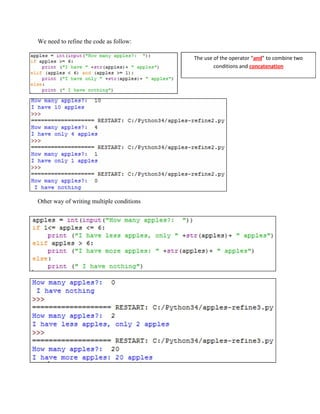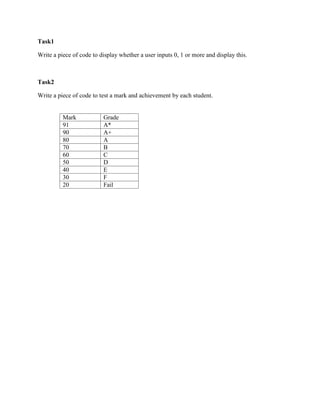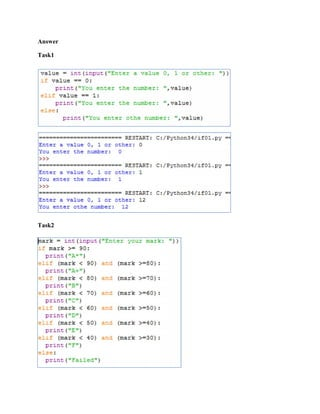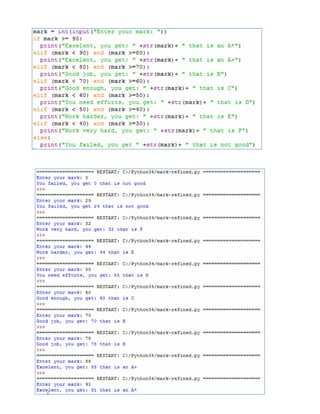Anzeige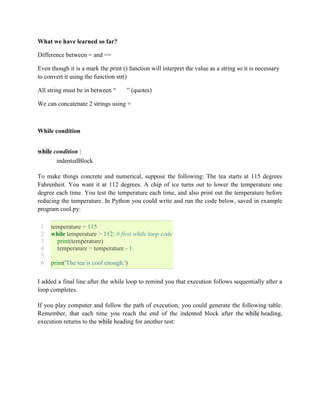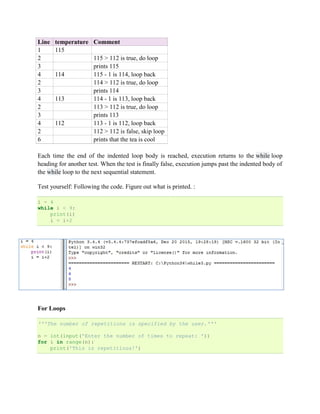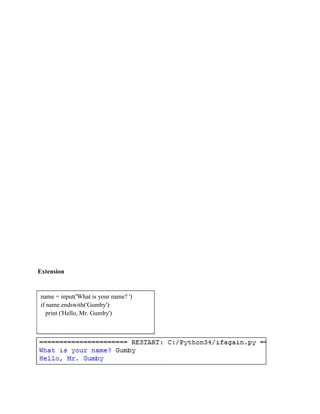Nächste SlideShare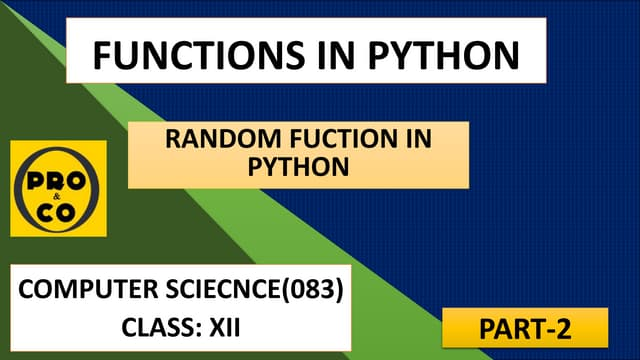FUNCTIONS IN PYTHON[RANDOM FUNCTION]
1 von 17
Anzeige

### Introduction to python programming

1. COMPUTER STUDIES INTRODUCTION TO PYTHON PROGRAMMING Python programming is pretty straight forward. >>> 2 + 3 * 6 20 >>> (2 + 3) * 6 30 >>> 48565878 * 578453 28093077826734 >>> 2 ** 8 256 >>> 23 / 7 3.2857142857142856 >>> 23 // 7 3 >>> 23 % 7 2 >>> 2 + 2 4 >>> (5 - 1) * ((7 + 1) / (3 - 1)) 16.0 Order of operation: B (Brackets) E (Exponent) D (Division) M (Multiplication) A (Addition) S (Subtraction) Comments: # this is the first comment spam = 1 # and this is the second comment # ... and now a third! text = "# This is not a comment because it's inside quotes." Single line comment: # this is a single line comment Block comments “”” this is a block comments that we must put in a 3 double quote Like this”””
2. Basic Arithmetic Operations Operators: +: Addition -: Subtraction *: Multiplication /: Division %: Modulo (remainder). The remainder can be calculated with the % operator **: Power //: floor division: if both operands are of type int, floor division is performed and an int is returned Variables Variable = 18 Variable1 = “Hello world!” Types of variables:  int: integer 2, 4, 20  float: the ones with a fractional part or decimal (3.4, 4.9, 3.14,…)  complex:  bool: Boolean value (Yes/No; True/False; 0/1)  str: string this is a strings of characters “I am a string of characters” Data types: Numbers, Strings, Lists, Tuples, Dictionaries, Lists Functions In the context of programming, a function is a named sequence of statements that performs a desired operation. This operation is specified in a function definition. In Python, the syntax for a function definition is: print(), input(), round()
3. Built-in functions Numbers Using Python as a Calculator tax = 12.5 / 100 price = 100.50 bill = price * tax pay = round(bill, 2) print(“Your final bil is: “,pay,”\$”) The function round () (like in excel) rounds the result pay into 2 decimal places
4. Strings Besides numbers, Python can also manipulate strings, which can be expressed in several ways. They can be enclosed in single quotes ('...') or double quotes ("...") with the same result . can be used to escape quotes: >>> 'spam eggs' # single quotes 'spam eggs' >>> 'doesn't' # use ' to escape the single quote... "doesn't" >>> "doesn't" # ...or use double quotes instead "doesn't" >>> '"Yes," he said.' '"Yes," he said.' >>> ""Yes," he said." '"Yes," he said.' >>> '"Isn't," she said.' '"Isn't," she said.' Concatenate strings Prefix = “Py” Word = Prefix + “thon” Lists Built-in function: len()
5. Manipulate the element in the list. Index element in the list The other way around, if we want to know the index of the element in the list we can use: This program will return the index of the element “horse” in the list that is 2 Modify, append elements in the list Let’s modify (replace) “bull” with “parrot” Bull is replaced by parrot
6. Extend the list and append elements If we extend the list the new element is added at the end of the list How if we move the “bull” at the beginning of the list Bull is moved at the beginning of the list Bull is added again in the list
7. Assignment to slices is also possible, and this can even change the size of the list or clear it entirely It is possible to nest lists (create lists containing other lists)
8. In x= [a, n] The first element of the list must be a in that case x =”a” and the second element must be x = n If we want to show the elements of the list in a it must be written as: x[0,1,2] Instead for the element from n, it is: x[0,1,2,3] Et voilà c’est fait!
9. The elements of X = [a, b, c] So from the figure above: X   = a; X   = b; X   = c The elements of X  = [1, 2, 3] So from the figure above: X   = 1; X   = 2; X   = 3 x [ a , n= ] First element X  Second element X       
10. Conditions and Conditional Statements if, else, elif (Elseif) <, >, <=, >=, ==, != # and, or, not, !=, == if 12!=13 print (“YES") a = 33 t = 22i if a == 33 and not (t ==22): print ("TRUE") else: print("FALSE") apples = input("How many apples?") if apples >= 6: print ("I have more apples: ") elif apples < 6: print ("I have not enough apples: ") else: print (" I have nothing: ") apples = int(input("How many apples?: ")) if apples >= 6: print ("I have more apples: ",str(apples)) elif apples < 6: print ("I have not enough apples: ",str(apples)) else: print (" I have nothing: ",str(apples)) It should say, “I have nothing” We try to compare string and integer so we need to convert it into integer using the function int ()
11. We need to refine the code as follow: Other way of writing multiple conditions The use of the operator “and” to combine two conditions and concatenation
12. Task1 Write a piece of code to display whether a user inputs 0, 1 or more and display this. Task2 Write a piece of code to test a mark and achievement by each student. Mark Grade 91 A* 90 A+ 80 A 70 B 60 C 50 D 40 E 30 F 20 Fail